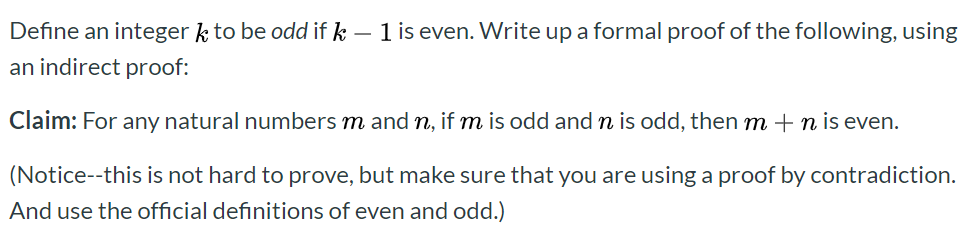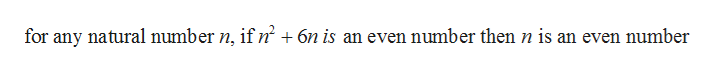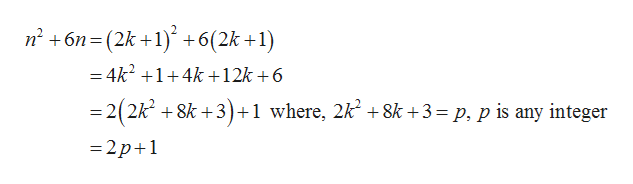# Define an integer k to be odd if k 1 is even. Write up a formal proof of the following, usingan indirect proof:Claim: For any natural numbers m and n, if m is odd and n is odd, then mn is even.(Notice--this is not hard to prove, but make sure that you are using a proof by contradiction.And use the official definitions of even and odd.)

Question
6 views

Prove the following:

For any natural number n, if n 2 + 6 n is an even number, then n is an even number.help_outlineImage TranscriptioncloseDefine an integer k to be odd if k 1 is even. Write up a formal proof of the following, using an indirect proof: Claim: For any natural numbers m and n, if m is odd and n is odd, then m n is even. (Notice--this is not hard to prove, but make sure that you are using a proof by contradiction. And use the official definitions of even and odd.) fullscreen
check_circle

Step 1

Consider the following information and prove the following:help_outlineImage Transcriptionclosefor any natural number n, if n' +6n is an even number then n is an even number fullscreen
Step 2

Prove the above result by contradiction:

Since, if n2+6n is an even number then n is an even number.

Let n is an odd number so, n=2k+1

So,help_outlineImage Transcriptionclosen26n (2k 1) +6(2k +1) =4k2 +1+4k +12k +6 2(2k2 8k +3)+1 where, 2k2 8k +3 = p, p is any integer =2p+1 fullscreen
Step 3

This leads us to a contradiction because n2+6n is an even number but from the assumption it came out to be...

### Want to see the full answer?

See Solution

#### Want to see this answer and more?

Solutions are written by subject experts who are available 24/7. Questions are typically answered within 1 hour.*

See Solution
*Response times may vary by subject and question.
Tagged in

### Math